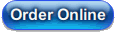# Bayesian Econometrics Course

This workbook is based upon the content of the RATS e-course on Bayesian Econometrics, offered in spring 2009. It covers most of the most important methods now used in Bayesian analysis in econometrics, including Gibbs sampling, Metropolis-Hastings and importance sampling. The applications are to a broad range of topics, include time series, cross-section and panel data. It assumes that the user is comfortable with such basic instructions as COMPUTE, DISPLAY, GRAPH, SCATTER and LINREG, and can use simple programming techniques such as DO loops. In each chapter, there is a Tips and Tricks section which covers in greater detail any functions or instructions that might be unfamiliar.

The presentation is based largely on Gary Koop's Bayesian Econometrics (Koop (2003)). We've added to that in several areas, with a chapter on vector autoregressions, and examples from the literature for panel, cross-sectional data and state-space models. In most cases, we've included much of the statistical derivations from the book, presented in a way to highlight the calculations as they are done with RATS, so even those without the book can benefit.

### Workbook Contents

(194 pages, 30 examples)

#### 1 Introduction

1.1 Bayesian Statistics: An Overview
1.2 Single Parameter--Brute Force
1.3 RATS Tips and Tricks
Example 1.1 Brute Force: Analyzing on a Grid

#### 2 Linear Regression Model with Conjugate Prior

2.1 LRM with a Single Variable
2.2 Normal Linear Model: Theory
2.3 Using Cross Product Matrices
2.4 Calculations
2.5 Simulations
2.6 RATS Tips and Tricks
Example 2.1 Linear Model: Single Variable
Example 2.2 Multiple Regression: Conjugate Prior
Example 2.3 Multiple Regression with Conjugate Prior: Simulations

#### 3 Normal Linear Model with Independent Prior

3.1 Theory
3.2 Calculations
3.3 Diagnostics
3.4 The Bayesian Approach to Hypothesis Testing
3.5 Hypothesis Testing with the Linear Model
3.6 RATS Tips and Tricks
Example 3.1 Linear Model with Independent Prior
Example 3.2 Linear Regression: Conjugate Prior with Restrictions

#### 4 Nonlinear Regression: Introduction to Metropolis-Hastings

4.1 Theory
4.2 Calculations
4.3 RATS Tips and Tricks
Example 4.1 Non-linear Regression: Random Walk MH
Example 4.2 Non-linear Regression: Independence MH

#### 5 Linear Regression with Non-Spherical Errors

5.1 Heteroscedasticity of Known Form
5.2 Heteroscedasticity of Unknown Form
5.3 Serially Correlated Errors
5.4 Seemingly Unrelated Regressions
5.5 RATS Tips and Tricks
Example 5.1 Heteroscedastic errors with a known form
Example 5.2 Heteroscedastic errors with a unknown functional form
Example 5.3 Linear regression with AR(1) errors
Example 5.4 Seemingly unrelated regression

#### 6 Vector Autoregressions

6.1 Flat Prior
6.2 Antithetic Acceleration
6.3 An Application: Blanchard-Quah Model
6.4 Structural VAR's
6.5 Informative Prior for Univariate Autoregressions
6.6 VAR with Informative Prior (BVAR)
6.7 RATS Tips and Tricks
Example 6.1 Antithetic Acceleration
Example 6.2 VAR with flat prior
Example 6.3 Structural VAR: Importance sampling
Example 6.4 Structural VAR: Random Walk MH
Example 6.5 Structural VAR: Independence Chain MH
Example 6.6 Univariate autoregression with prior
Example 6.7 Univariate Autoregression: Out-of-sample forecast performance
Example 6.8 Bayesian VAR: Gibbs sampling

#### 7 Cross Section and Panel Data

7.1 Panel Data
7.2 Probit and Tobit Models
7.3 RATS Tips and Tricks
Example 7.1 Panel data: LSDV
Example 7.2 Panel data: Fixed Effects
Example 7.3 Panel data: Random Effects (hierarchical prior)
Example 7.4 Panel data: Random coefficients model
Example 7.5 Tobit model
Example 7.6 Probit model

#### 8 State Space Models

Example 8.1 State-space model: Independence MH
Example 8.2 State Space Model: Gibbs sampling
Example 8.3 State space model: Time-varying coefficients

#### B Probability Distributions

B.1 Uniform
B.2 Univariate Normal
B.3 Univariate Student (t)
B.4 Beta distribution
B.5 Gamma Distribution
B.6 Inverse Gamma Distribution
B.7 Bernoulli Distribution
B.8 Multivariate Normal
B.9 Multivariate Student (t)
B.10 Wishart Distribution